## Design MPC Controller for Position Servomechanism

This example shows how to design a model predictive controller for a position servomechanism using MPC Designer.

### System Model

A position servomechanism consists of a DC motor, gearbox, elastic shaft, and load.The differential equations representing this system are

`$\begin{array}{l}{\stackrel{˙}{\omega }}_{L}=-\frac{{k}_{T}}{{J}_{L}}\left({\theta }_{L}-\frac{{\theta }_{M}}{\rho }\right)-\frac{{\beta }_{L}}{{J}_{L}}{\omega }_{L}\\ {\stackrel{˙}{\omega }}_{M}=\frac{{k}_{M}}{{J}_{M}}\left(\frac{V-{k}_{M}{\omega }_{M}}{R}\right)-\frac{{\beta }_{M}{\omega }_{M}}{{J}_{M}}+\frac{{k}_{T}}{\rho {J}_{M}}\left({\theta }_{L}-\frac{{\theta }_{M}}{\rho }\right)\end{array}$`

where,

• V is the applied voltage.

• T is the torque acting on the load.

• ${\omega }_{L}={\stackrel{˙}{\theta }}_{L}$ is the load angular velocity.

• ${\omega }_{M}={\stackrel{˙}{\theta }}_{M}$ is the motor shaft angular velocity.

The remaining terms are constant parameters.

Constant Parameters for Servomechanism Model

Symbol

Value (SI Units)

Definition

kT

1280.2

Torsional rigidity

kM

10

Motor constant

JM

0.5

Motor inertia

JL

50JM

ρ

20

Gear ratio

βM

0.1

Motor viscous friction coefficient

βL

25

R

20

Armature resistance

If you define the state variables as

`${x}_{p}={\left[\begin{array}{cccc}{\theta }_{L}& {\omega }_{L}& {\theta }_{M}& {\omega }_{M}\end{array}\right]}^{T},$`

then you can model the servomechanism as an LTI state-space system.

`$\begin{array}{c}{\stackrel{˙}{x}}_{p}=\left[\begin{array}{cccc}0& 1& 0& 0\\ -\frac{{k}_{T}}{{J}_{L}}& -\frac{{\beta }_{L}}{{J}_{L}}& \frac{{k}_{T}}{\rho {J}_{L}}& 0\\ 0& 0& 0& 1\\ \frac{{k}_{T}}{\rho {J}_{M}}& 0& -\frac{{k}_{T}}{{\rho }^{2}{J}_{M}}& -\frac{{\beta }_{M}+\frac{{k}_{M}^{2}}{R}}{{J}_{M}}\end{array}\right]{x}_{p}+\left[\begin{array}{c}0\\ 0\\ 0\\ \frac{{k}_{M}}{R{J}_{M}}\end{array}\right]V\\ {\theta }_{L}=\left[\begin{array}{cccc}1& 0& 0& 0\end{array}\right]{x}_{p}\\ T=\left[\begin{array}{cccc}{k}_{T}& 0& -\frac{{k}_{T}}{\rho }& 0\end{array}\right]{x}_{p}\end{array}$`

The controller must set the angular position of the load, θL, at a desired value by adjusting the applied voltage, V.

However, since the elastic shaft has a finite shear strength, the torque, T, must stay within the range |T| ≤ 78.5 Nm. Also, the voltage source physically limits the applied voltage to the range |V| ≤ 220 V.

### Construct Plant Model

Specify the model constants.

```Kt = 1280.2; % Torsional rigidity Km = 10; % Motor constant Jm = 0.5; % Motor inertia Jl = 50*Jm; % Load inertia N = 20; % Gear ratio Bm = 0.1; % Rotor viscous friction Bl = 25; % Load viscous friction R = 20; % Armature resistance```

Define the state-space matrices derived from the model equations.

```A = [ 0 1 0 0; -Kt/Jl -Bl/Jl Kt/(N*Jl) 0; 0 0 0 1; Kt/(Jm*N) 0 -Kt/(Jm*N^2) -(Bm+Km^2/R)/Jm]; B = [0; 0; 0; Km/(R*Jm)]; C = [ 1 0 0 0; Kt 0 -Kt/N 0]; D = [0; 0]; ```

Create a state-space model.

`plant = ss(A,B,C,D);`

### Open MPC Designer App

`mpcDesigner`

### Import Plant and Define Signal Configuration

In MPC Designer, on the MPC Designer tab, select MPC Structure.

In the Define MPC Structure By Importing dialog box, select the `plant` plant model, and assign the plant I/O channels to the following signal types:

• Manipulated variable — Voltage, V

• Measured output — Load angular position, θL

• Unmeasured output — Torque, T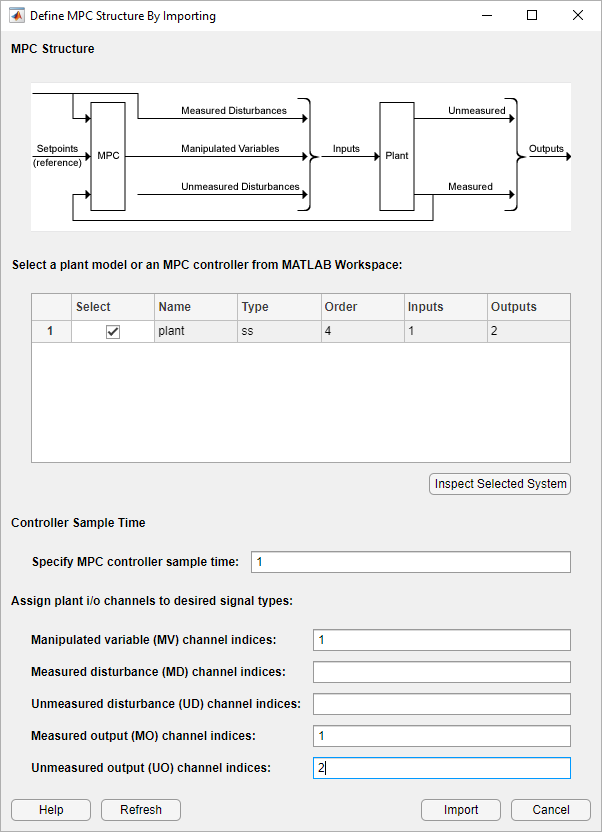Click .

MPC Designer imports the specified plant to the Data Browser. The following are also added to the Data Browser:

• `mpc1` — Default MPC controller created using `plant` as its internal model.

• `scenario1` — Default simulation scenario. The results of this simulation are displayed in the Input Response and Output Response plots.

### Define Input and Output Channel Attributes

On the MPC Designer tab, in the Structure section, click I/O Attributes.

In the Input and Output Channel Specifications dialog box, for each input and output channel:

• Specify a meaningful Name and Unit.

• Keep the Nominal Value at its default value of `0`.

• Specify a Scale Factor for normalizing the signal. Select a value that approximates the predicted operating range of the signal:

Channel NameMinimum ValueMaximum ValueScale Factor
`Voltage`–220 V220 V`440`
`Theta`–π radiansπ radians`6.28`
`Torque`–78.5 Nm78.5 Nm`157`Click to update the channel attributes and close the dialog box.

### Modify Scenario To Simulate Angular Position Step Response

In the Scenario section, Edit Scenario drop-down list, select `scenario1` to modify the default simulation scenario.

In the Simulation Scenario dialog box, specify a Simulation duration of `10` seconds.

In the Reference Signals table, keep the default configuration for the first channel. These settings create a `Step` change of `1` radian in the angular position setpoint at a Time of `1` second.

For the second output, in the Signal drop-down list, select `Constant` to keep the torque setpoint at its nominal value.Click .

The app runs the simulation with the new scenario settings and updates the Input Response and Output Response plots.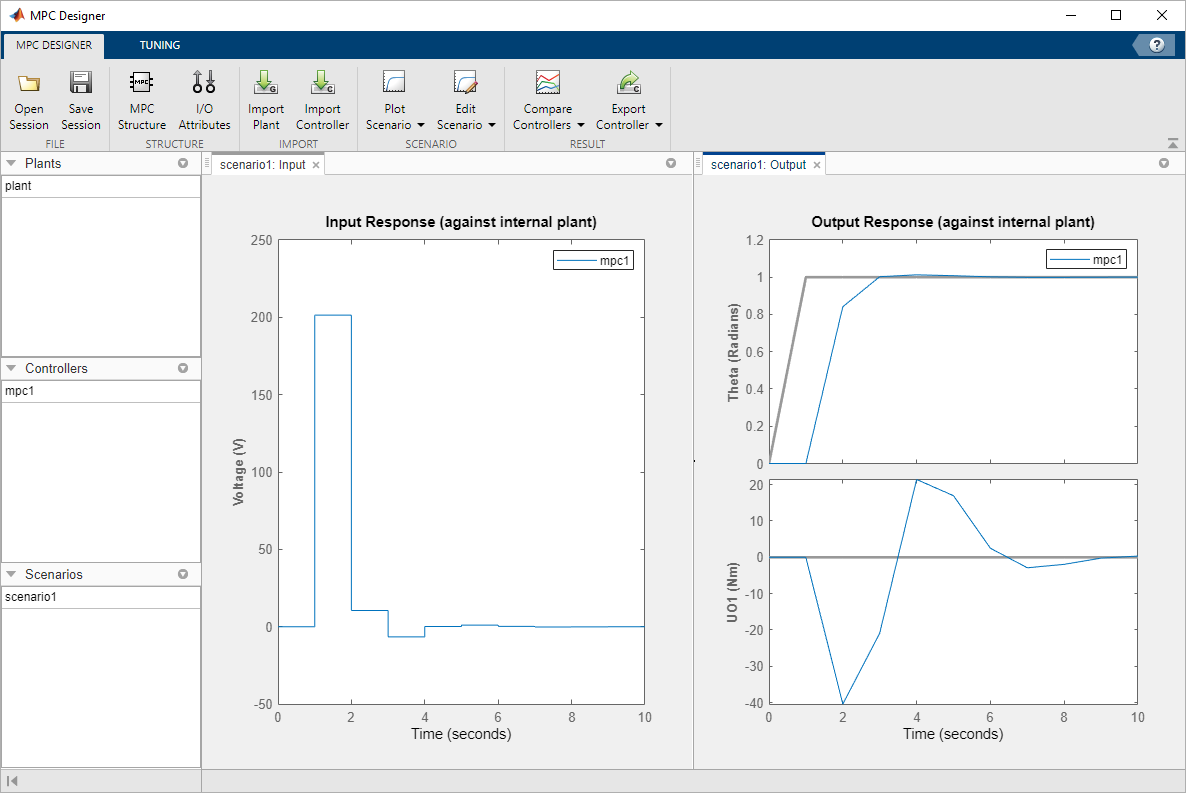### Specify Controller Sample Time and Horizons

On the Tuning tab, in the Horizon section, specify a Sample time of `0.1` seconds.

For the specified sample time, Ts, and a desired response time of Tr = 2 seconds, select a prediction horizon, p, such that:

`${T}_{r}\approx p{T}_{s}.$`

Therefore, specify a Prediction horizon of `20`.

Specify a Control horizon of `5`.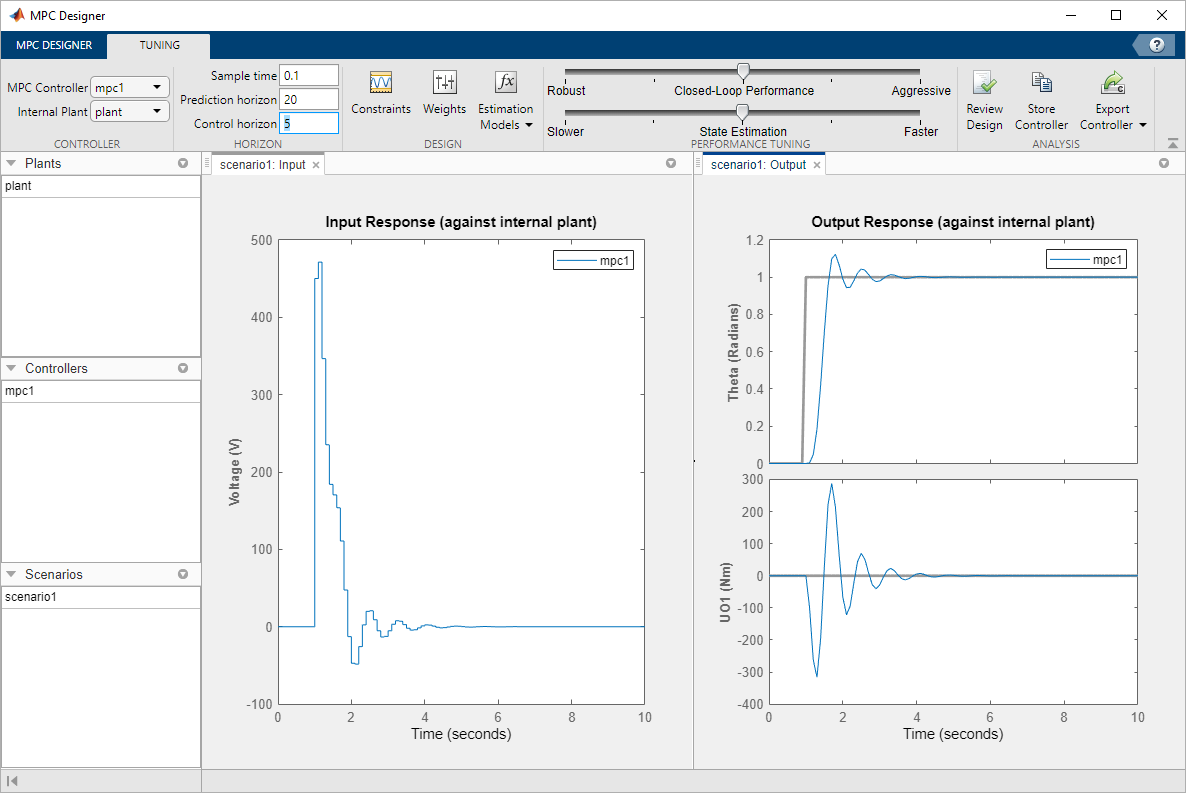As you update the sample time and horizon values, the Input Response and Output Response plots update automatically. Both the input voltage and torque values exceed the constraints defined in the system model specifications.

### Specify Constraints

In the Design section, select Constraints.

In the Constraints dialog box, in the Input Constraints section, specify the Min and Max voltage values for the manipulated variable (MV).

In the Output Constraints section, specify Min and Max torque values for the unmeasured output (UO).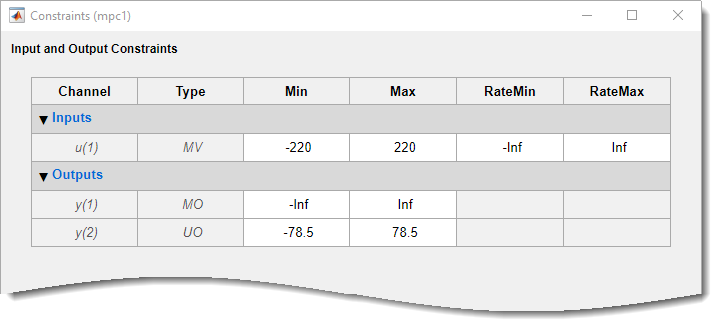There are no additional constraints, that is the other constraints remain at their default maximum and minimum values, `—Inf` and `Inf` respectively

Click .The response plots update to reflect the new constraints. In the Input Response plot, there are undesirable large changes in the input voltage.

### Specify Tuning Weights

In the Design section, select Weights.

In the Weights dialog box, in the Input Weights table, increase the manipulated variable Rate Weight.The tuning Weight for the manipulated variable (MV) is `0`. This weight indicates that the controller can allow the input voltage to vary within its constrained range. The increased Rate Weight limits the size of manipulated variable changes.

Since the control objective is for the angular position of the load to track its setpoint, the tuning Weight on the measured output is `1`. There is no setpoint for the applied torque, so the controller can allow the second output to vary within its constraints. Therefore, the Weight on the unmeasured output (UO) is `0`, which enables the controller to ignore the torque setpoint.

Click .The response plots update to reflect the increased rate weight. The Input Response is smoother with smaller voltage changes.

### Examine Output Response

In the Output Response plot, right-click the Theta plot area, and select Characteristics > Peak Response.The peak output response occurs at time of 3 seconds with a maximum overshoot of 3%. Since the reference signal step change is at 1 second, the controller has a peak time of 2 seconds.

### Improve Controller Response Time

Click and drag the Closed-Loop Performance slider to the right to produce a more Aggressive response. The further you drag the slider to the right, the faster the controller responds. Select a slider position such that the peak response occurs at 2.7 seconds.The final controller peak time is 1.7 seconds. Reducing the response time further results in overly-aggressive input voltage changes.

### Generate and Run MATLAB Script

In the Analysis section, click the Export Controller arrow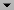.

Under Export Controller, click ```Generate Script```.

In the Generate MATLAB® Script dialog box, check the box next to `scenario1`.

Click .

The app exports a copy of the plant model, `plant_C`, to the MATLAB workspace, along with simulation input and reference signals.

Additionally, the app generates the following code in the MATLAB Editor.

```%% create MPC controller object with sample time mpc1 = mpc(plant_C, 0.1); %% specify prediction horizon mpc1.PredictionHorizon = 20; %% specify control horizon mpc1.ControlHorizon = 5; %% specify nominal values for inputs and outputs mpc1.Model.Nominal.U = 0; mpc1.Model.Nominal.Y = [0;0]; %% specify scale factors for inputs and outputs mpc1.MV(1).ScaleFactor = 440; mpc1.OV(1).ScaleFactor = 6.28; mpc1.OV(2).ScaleFactor = 157; %% specify constraints for MV and MV Rate mpc1.MV(1).Min = -220; mpc1.MV(1).Max = 220; %% specify constraints for OV mpc1.OV(2).Min = -78.5; mpc1.OV(2).Max = 78.5; %% specify overall adjustment factor applied to weights beta = 1.2712; %% specify weights mpc1.Weights.MV = 0*beta; mpc1.Weights.MVRate = 0.4/beta; mpc1.Weights.OV = [1 0]*beta; mpc1.Weights.ECR = 100000; %% specify simulation options options = mpcsimopt(); options.RefLookAhead = 'off'; options.MDLookAhead = 'off'; options.Constraints = 'on'; options.OpenLoop = 'off'; %% run simulation sim(mpc1, 101, mpc1_RefSignal, mpc1_MDSignal, options);```

In the MATLAB Window, in the Editor tab, select Save.

Complete the Save dialog box and then click .

In the Editor tab, click Run.The script creates the controller, `mpc1`, and runs the simulation scenario. The input and output responses match the simulation results from the app.

### Validate Controller Performance In Simulink

If you have a Simulink® model of your system, you can simulate your controller and validate its performance. Simulink functionality is not supported in MATLAB Online™.

Open the model.

`open_system('mpc_motor')`This model uses an MPC Controller block to control a servomechanism plant. The Servomechanism Model block is already configured to use the `plant` model from the MATLAB workspace.

The Angle reference source block creates a sinusoidal reference signal with a frequency of `0.4` rad/sec and an amplitude of π.

Double-click the MPC Controller block.

In the MPC Controller Block Parameters dialog box, specify an MPC Controller from the MATLAB workspace. Use the `mpc1` controller created using the generated script.Click .

At the MATLAB command line, specify a torque magnitude constraint variable.

`tau = 78.5;`

The model uses this value to plot the constraint limits on the torque output scope.

In the Simulink model window, click Run to simulate the model.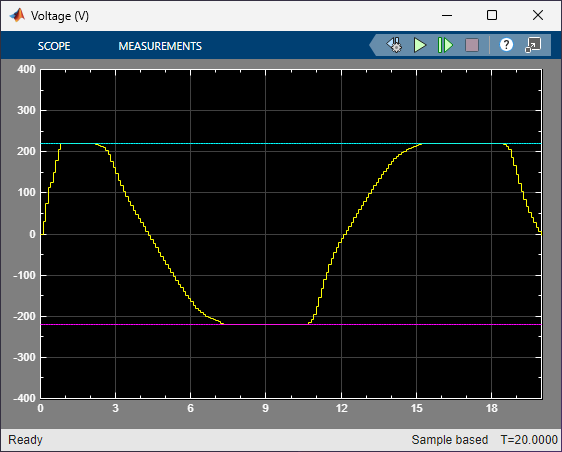In the Angle scope, the output response, yellow, tracks the angular position setpoint, blue, closely.